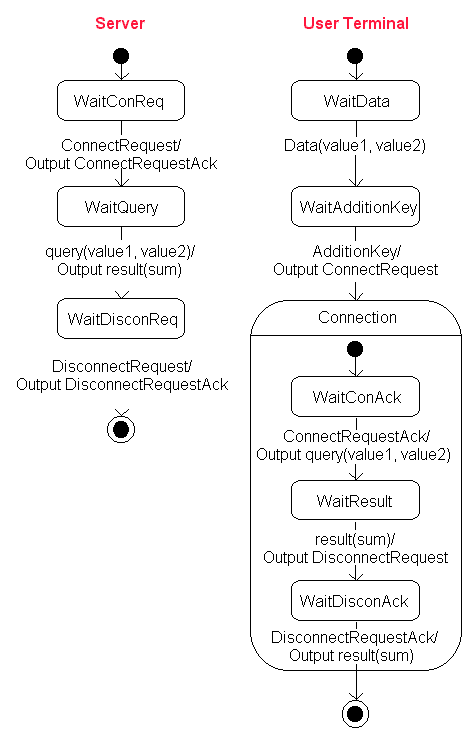# Definition: UML

(Unified Modeling Language) An object-oriented analysis and design language from the Object Management Group (OMG). Many design methodologies for describing object-oriented systems were developed in the late 1980s. UML standardizes several diagramming methods, including Grady Booch's work at Rational Software, Rumbaugh's Object Modeling Technique and Ivar Jacobson's work on use cases.

There are twelve diagrams supported under UML. Four are structural, five are behavioral and three are used for model management, which include packages, subsystems and models. See XMI.

Structural Diagrams
The four structural diagrams are class, object, component and deployment. Following is an example of the class diagram:Class Diagram
This is one of the structural diagrams. User, User Terminal and Server are three classes that have attributes and operations. An actor is a UML "stereotype" that is external to the system. Lines between classes are "associations." The asterisk means "many," in this case, a many-to-one relationship between client and server.

Behavioral Diagrams
There are five behavioral diagrams: use case, sequence, activity, collaboration and state chart. Following are examples of all but the activity diagram:Use Case Diagram
This diagram shows a system's functions from a user's point of view, which in this case is pretty simple. All the following diagrams were created in the Telelogic Tau UML suite by Telelogic for this same example. Five of the nine possible diagrams available in UML are shown below. The Component Diagram (software module interaction), Deployment Diagram (hardware nodes) and the Activity Diagram (tasks) are not included. The Object Diagram (instantiations of classes) is rarely used. (All diagrams courtesy of Telelogic, AB, www.telelogic.com)Sequence Diagram
Like an MSC (Message Sequence Diagram), the Sequence Diagram depicts the message flow between entities in the system. The items between double left/right arrows are UML "stereotypes." See MSC.Collaboration Diagram
This diagram emphasizes the structure of the relationships between entities. Note that the user is associated with the user terminal in a session, and the terminal is associated with the server in a connection. The order of messages can be read by interpreting the numbers prefixes.State Chart
This describes the finite states that take place in the system. The rectangles are the states, and the lines between them are the transitions. The connection state shows three substates. The single circles are starting points, and the double circles are the ends.